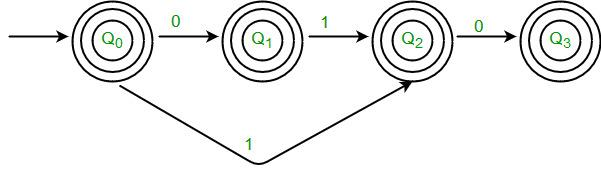Courses

# Test: Automata

## 10 Questions MCQ Test Theory of Computation | Test: Automata

Description
This mock test of Test: Automata for Computer Science Engineering (CSE) helps you for every Computer Science Engineering (CSE) entrance exam. This contains 10 Multiple Choice Questions for Computer Science Engineering (CSE) Test: Automata (mcq) to study with solutions a complete question bank. The solved questions answers in this Test: Automata quiz give you a good mix of easy questions and tough questions. Computer Science Engineering (CSE) students definitely take this Test: Automata exercise for a better result in the exam. You can find other Test: Automata extra questions, long questions & short questions for Computer Science Engineering (CSE) on EduRev as well by searching above.
QUESTION: 1

### Assume the R is a relation on a set A, aRb is partially ordered such that a and b are _____________

Solution:

A partially ordered relation refers to one which is Reflexive, Transitive and Antisymmetric.

QUESTION: 2

### The non- Kleene Star operation accepts the following string of finite length over set A = {0,1} | where string s contains even number of 0 and 1

Solution:

The Kleene star of A, denoted by A*, is the set of all strings obtained by concatenating zero or more strings from A.

QUESTION: 3

### A regular language over an alphabet ∑ is one that cannot be obtained from the basic languages using the operation

Solution:

Union, Intersection, Concatenation, Kleene*, Reverse are all the closure properties of Regular Language.

QUESTION: 4

Statement 1: A Finite automata can be represented graphically; Statement 2: The nodes can be its states; Statement 3: The edges or arcs can be used for transitions
Hint: Nodes and Edges are for trees and forests too.
Which of the following make the correct combination?

Solution:

It is possible to represent a finite automaton graphically, with nodes for states, and arcs for transitions.

QUESTION: 5

The minimum number of states required to recognize an octal number divisible by 3 are/is

Solution:

According to the question, minimum of 3 states are required to recognize an octal number divisible by 3.

QUESTION: 6

Which of the following is a not a part of 5-tuple finite automata?

Solution:

Explanation: A FA can be represented as FA= (Q, ∑, δ, q0, F) where Q=Finite Set of States, ∑=Finite Input Alphabet, δ=Transition Function, q0=Initial State, F=Final/Acceptance State).

QUESTION: 7

If an Infinite language is passed to Machine M, the subsidiary which gives a finite solution to the infinite input tape is ______________

Solution:

A Compiler is used to give a finite solution to an infinite phenomenon. Example of an infinite phenomenon is Language C, etc.

QUESTION: 8

The number of elements in the set for the Language L={xϵ(∑r) *|length if x is at most 2} and ∑={0,1} is_________

Solution:

∑r= {1,0} and a Kleene* operation would lead to the following set=COUNT{ε,0,1,00,11,01,10} =7.

QUESTION: 9

Let w be any string of length n is {0,1}*. Let L be the set of all substrings of w. What is the minimum number of states in a non-deterministic finite automaton that accepts L?

Solution:

We need minimum n+1 states to build NFA that accepts all substrings of a binary string. For example, following NFA accepts all substrings of “010″ and it has 4 states.QUESTION: 10

Given: ∑= {a, b}
L= {xϵ∑*|x is a string combination}
∑4 represents which among the following?

Solution:

Explanation: ∑* represents any combination of the given set while ∑x represents the set of combinations with length x where x ϵ I.